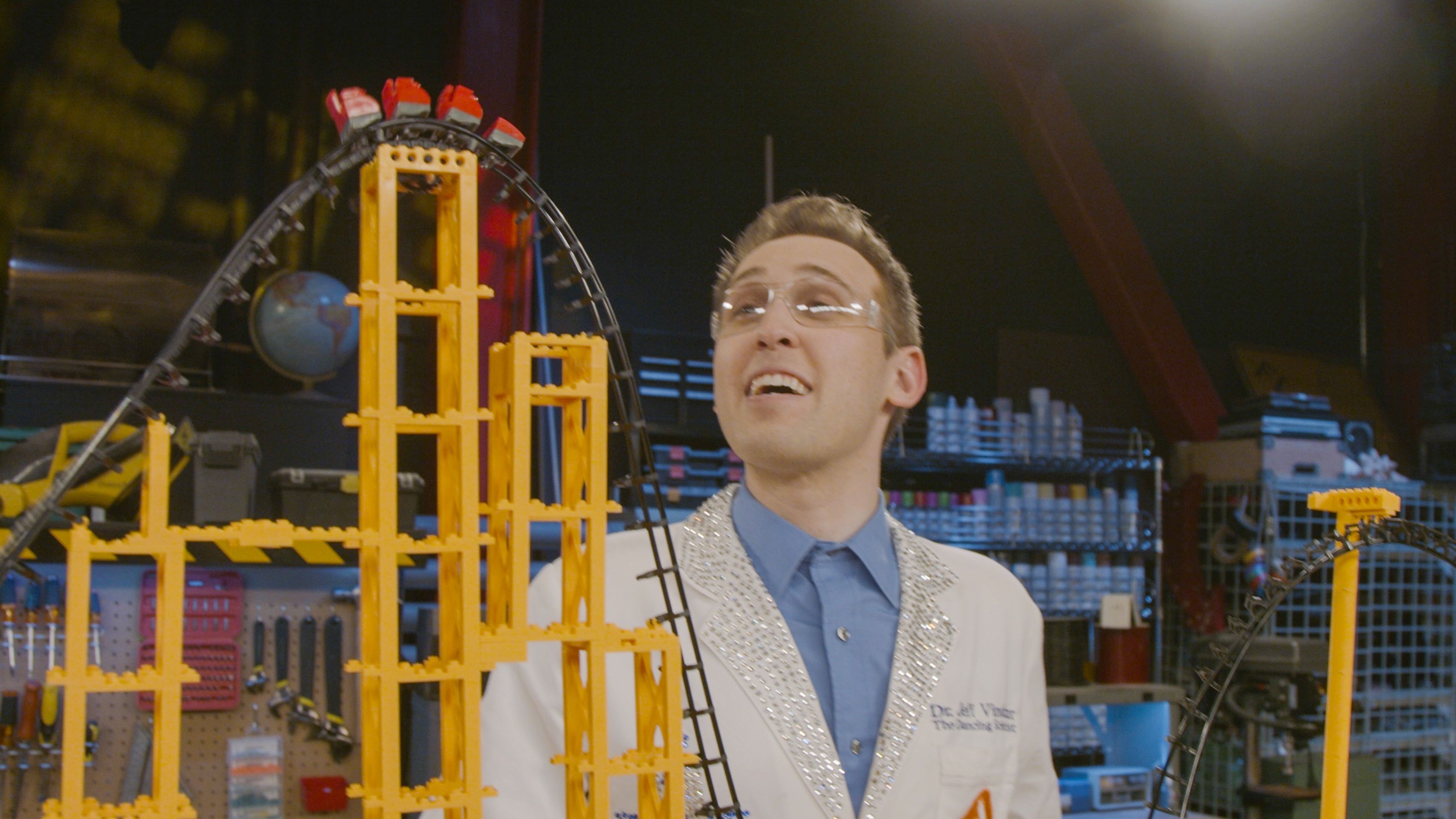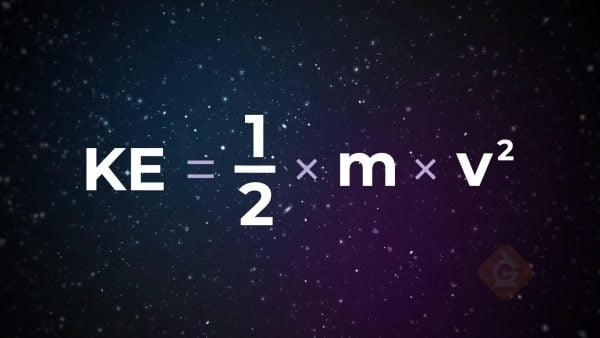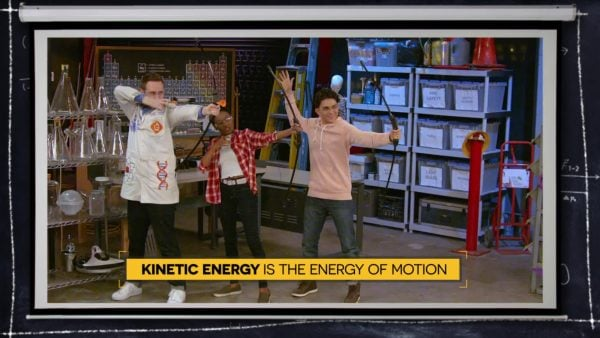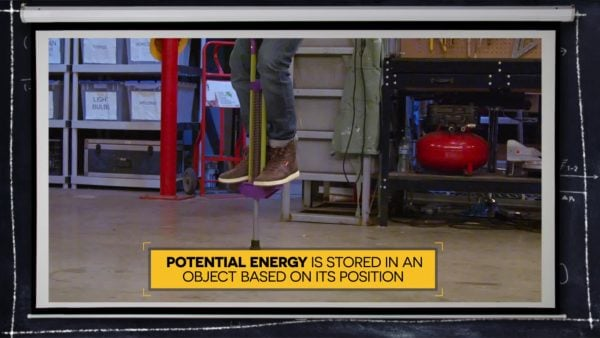Read About Potential & Kinetic Energy | Science for Grades 6-8 [Printable]
1%
It was processed successfully!WHAT IS POTENTIAL VS. KINETIC ENERGY?

Energy makes stuff happen. Energy associated with the motion of an object is called kinetic energy. Energy stored in an object due to its position is called potential energy. Energy can be converted between potential and kinetic based on an object’s motion.

To better understand Potential vs. Kinetic Energy…

WHAT IS POTENTIAL VS. KINETIC ENERGY?. Energy makes stuff happen. Energy associated with the motion of an object is called kinetic energy. Energy stored in an object due to its position is called potential energy. Energy can be converted between potential and kinetic based on an object’s motion. To better understand Potential vs. Kinetic Energy…

## LET’S BREAK IT DOWN!

### Energy makes stuff happen.Richard Feynman, one of the greatest physicists of the twentieth century, told his students that, “It is important to realize that, in physics today, we have no knowledge of what energy is. We do not have a picture that energy comes in little blobs of a definite amount. It is not that way. However, there are formulas for calculating some numerical quantity, and when we add it all together it gives … always the same number. It is an abstract thing in that it does not tell us the mechanism or the reasons for the various formulas“(Feynman, Leighton, and Sands 1965, p. 4-2). According to Feynman, it is more important to describe how energy behaves in a system than to define what energy is. One thing we know is that energy makes stuff happen, and we can calculate it very precisely when it does.

Energy makes stuff happen. Richard Feynman, one of the greatest physicists of the twentieth century, told his students that, “It is important to realize that, in physics today, we have no knowledge of what energy is. We do not have a picture that energy comes in little blobs of a definite amount. It is not that way. However, there are formulas for calculating some numerical quantity, and when we add it all together it gives … always the same number. It is an abstract thing in that it does not tell us the mechanism or the reasons for the various formulas“(Feynman, Leighton, and Sands 1965, p. 4-2). According to Feynman, it is more important to describe how energy behaves in a system than to define what energy is. One thing we know is that energy makes stuff happen, and we can calculate it very precisely when it does.

### Energy associated with the motion of an object is called kinetic energy (KE).From your youngest age, you probably associated motion with energy. You intuitively knew that the faster something moved, the more energy it must have. A more precise term for energy of motion is kinetic energy. The amount of KE an object has depends upon its mass and its velocity (speed in a given direction). By making many observations over time, scientists determined that the KE of an object is equal to one-half its mass (m) times its velocity (v) squared, and they write the equation as:

KE = ½ × m × v²

The equation shows that KE is directly proportional to the mass of an object (KE ∝ m). As this graph shows, if we double the KE, then we double the mass, or if we halve the KE, then we halve the mass. It also shows that the relationship is linear (directly proportional, straight-line). That’s why wrecking balls are so big and heavy—more KE to destroy stuff!

Look at the relationship between KE and velocity shown in this graph. Again, we see that KE and velocity are directly proportional (KE ∝ v²), but if we increase the KE by a factor of 4, then the velocity of the object doubles (that’s the velocity times itself, not times 2). The graphed relationship is exponential (directly proportional where one of the variables is an exponent, curved line). Think of the damage a railgun can do shooting out a lightweight projectile at six thousand miles per hour without using explosives!

Energy associated with the motion of an object is called kinetic energy (KE). From your youngest age, you probably associated motion with energy. You intuitively knew that the faster something moved, the more energy it must have. A more precise term for energy of motion is kinetic energy. The amount of KE an object has depends upon its mass and its velocity (speed in a given direction). By making many observations over time, scientists determined that the KE of an object is equal to one-half its mass (m) times its velocity (v) squared, and they write the equation as: KE = ½ × m × v² The equation shows that KE is directly proportional to the mass of an object (KE ∝ m). As this graph shows, if we double the KE, then we double the mass, or if we halve the KE, then we halve the mass. It also shows that the relationship is linear (directly proportional, straight-line). That’s why wrecking balls are so big and heavy—more KE to destroy stuff! Look at the relationship between KE and velocity shown in this graph. Again, we see that KE and velocity are directly proportional (KE ∝ v²), but if we increase the KE by a factor of 4, then the velocity of the object doubles (that’s the velocity times itself, not times 2). The graphed relationship is exponential (directly proportional where one of the variables is an exponent, curved line). Think of the damage a railgun can do shooting out a lightweight projectile at six thousand miles per hour without using explosives!

### Energy stored in an object due to its position is called potential energy (PE).Where does the energy come from when an object is in motion? Let’s use a bow and arrow as an example to help us answer this question. You pull the arrow back and the bow bends. When you let go of the arrow, it flies through the air. The arrow got the energy for motion from the bend in the bow. The more you pull the arrow back, the more the bow bends, and the faster the arrow moves. The position of the bow determines how much energy the arrow will have. Energy stored in an object due to its position is called potential energy.

You’ve seen someone pantomime dropping a mic when they do something epic. Does the height from which the microphone is dropped have any effect on the amount of energy it has? Look at these three mics. One was dropped from a height of 1 meter, one from 2 meters, and one from 3 meters. The one dropped from 3 meters is shattered, which is evidence that an object higher from the ground has more potential energy.

Energy stored in an object due to its position is called potential energy (PE). Where does the energy come from when an object is in motion? Let’s use a bow and arrow as an example to help us answer this question. You pull the arrow back and the bow bends. When you let go of the arrow, it flies through the air. The arrow got the energy for motion from the bend in the bow. The more you pull the arrow back, the more the bow bends, and the faster the arrow moves. The position of the bow determines how much energy the arrow will have. Energy stored in an object due to its position is called potential energy. You’ve seen someone pantomime dropping a mic when they do something epic. Does the height from which the microphone is dropped have any effect on the amount of energy it has? Look at these three mics. One was dropped from a height of 1 meter, one from 2 meters, and one from 3 meters. The one dropped from 3 meters is shattered, which is evidence that an object higher from the ground has more potential energy.

### Energy can be converted between potential and kinetic based on an object’s motion.You probably noticed that in each of the examples (wrecking ball, railgun, bow and arrow, and mic drop), potential energy was converted to kinetic energy or kinetic energy was converted to potential energy. The arrow was pulled back (KE to pull; PE based on bend), then released (PE from position to KE as arrow moved through the air). Evidence that the bow and arrow system contained energy was the motion of the bow and the arrow, the sound the bow makes when the arrow is released, the sound the arrow makes as it moves through air and when it hits a target, and the heat your fingers feel on the string of the bow and on the target when the arrow strikes. Just like Feynman said, energy is hard to define, but we have evidence of it based on how the components of the system behave.

Energy can be converted between potential and kinetic based on an object’s motion. You probably noticed that in each of the examples (wrecking ball, railgun, bow and arrow, and mic drop), potential energy was converted to kinetic energy or kinetic energy was converted to potential energy. The arrow was pulled back (KE to pull; PE based on bend), then released (PE from position to KE as arrow moved through the air). Evidence that the bow and arrow system contained energy was the motion of the bow and the arrow, the sound the bow makes when the arrow is released, the sound the arrow makes as it moves through air and when it hits a target, and the heat your fingers feel on the string of the bow and on the target when the arrow strikes. Just like Feynman said, energy is hard to define, but we have evidence of it based on how the components of the system behave.

### Engineers solve a range of problems using their understanding of KE and PE.Many design problems require an understanding of kinetic and potential energy. How can we design a prosthetic leg so that the wearer can run faster? How can we make football helmets that protect a player’s head/brain when tackled? How can we improve safety features in an automobile that protect passengers during a collision? Mechanical engineers spend their careers solving these kinds of problems by applying their knowledge of kinetic and potential energy.

They are also the people who design the fabulous roller coasters at amusement parks. Their understanding of kinetic and potential energy allows them to make roller coasters thrilling but safe. Using the mass of the cart and how much energy may be lost as sound and heat energy as the cart moves along the track, mechanical engineers can determine how much potential energy the cart needs (how high the hill(s) need to be) to make it around a loop and to the end of the track.

Energy makes stuff happen! By understanding how it behaves in a system, we can explain and predict so many observations of the natural and designed world.

Source of quote: Feynman, R. P., R. B. Leighton, M. Sands, and E. M. Hafner. 1965. The Feynman lectures on physics, vol. 1. American Journal of Physics 33 (9): 750.

Engineers solve a range of problems using their understanding of KE and PE. Many design problems require an understanding of kinetic and potential energy. How can we design a prosthetic leg so that the wearer can run faster? How can we make football helmets that protect a player’s head/brain when tackled? How can we improve safety features in an automobile that protect passengers during a collision? Mechanical engineers spend their careers solving these kinds of problems by applying their knowledge of kinetic and potential energy. They are also the people who design the fabulous roller coasters at amusement parks. Their understanding of kinetic and potential energy allows them to make roller coasters thrilling but safe. Using the mass of the cart and how much energy may be lost as sound and heat energy as the cart moves along the track, mechanical engineers can determine how much potential energy the cart needs (how high the hill(s) need to be) to make it around a loop and to the end of the track. Energy makes stuff happen! By understanding how it behaves in a system, we can explain and predict so many observations of the natural and designed world. Source of quote: Feynman, R. P., R. B. Leighton, M. Sands, and E. M. Hafner. 1965. The Feynman lectures on physics, vol. 1. American Journal of Physics 33 (9): 750.

## POTENTIAL VS. KINETIC ENERGY VOCABULARY

Energy
Energy makes stuff happen; although it is hard to define energy, we can describe how it behaves in systems and how it is manifested in phenomena. (Disciplinary Core Ideas, pg. 60)
Kinetic Energy
Energy of motion.
Potential Energy
Energy stored in an object based on its position.
Gravity
Force of attraction between any two masses.
Energy Transfer
Process where energy is moved from one place to another.
Energy Conversion
Process where there is a change in energy from one form to another.
½ m X v2 (½ the mass times the velocity squared) which shows that the kinetic energy of an object is proportional to the mass and velocity of the object.
(In mathematics) having a constant ratio to another quantity.
Pattern of data that shows greater increase with passing time.

## POTENTIAL VS. KINETIC ENERGY DISCUSSION QUESTIONS

### What is the difference between kinetic and potential energy?

Kinetic energy is energy of motion and potential energy is associated with the relative position of something.

Mass and speed.

### What variable(s) determine the amount of potential energy an object has?

The object’s position and its mass.

### Explain how energy is transformed in a spring.

Potential energy is increased the more the spring is compressed. Once a person lets go of the spring, the potential energy is transformed to kinetic energy when the spring moves. (Students may also mention that some of the kinetic energy is converted to sound and heat.)

### Explain which has a greater impact on the kinetic energy of an object – the object’s mass or the object’s speed?

The speed of an object has a greater impact, because KE is proportional to ½ the mass of the object but to the speed squared.

### Explain how you could increase the potential energy of an object.

You could move the object to greater height or distance or you could increase an object’s mass.
X

## Success

We’ve sent you an email with instructions how to reset your password.
Ok

## Choose Your Free Trial Period### 7 DaysContinue to Lessons### 90 DaysGet 90 days free by inviting other teachers to try it too.

Share with Teachers

## Get 90 Days Free

### By inviting 4 other teachers to try it too.

4 required

Skip, I will use a 7 days free trial

## Thank You!

Enjoy your free 90 days trial